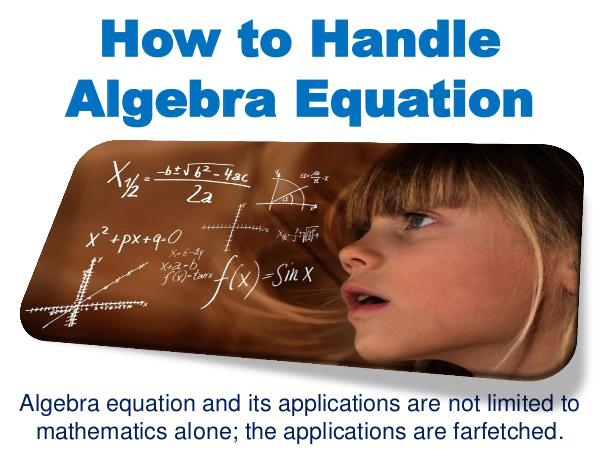点击阅读
• 评论

# How to Handle Algebra Equation1

Algebra equation and its applications are not limited to mathematics alone; the applications are farfetched. To say the fact, mathematicians are the real rulers of this present word. 更多
Algebra equation and its applications are not limited to mathematics alone; the applications are farfetched. To say the fact, mathematicians are the real rulers of this present word. 更多